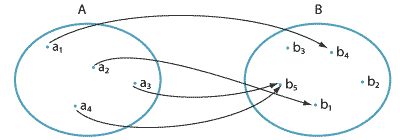### Functions between finite sets

If $$A$$ and $$B$$ are finite sets, then a function from $$A$$ to $$B$$ can be specified by listing all elements in $$A$$ and their images in $$B$$. For example, if

$A = \{a_1,a_2,a_3,a_4\} \qquad\text{and}\qquad B = \{b_1,b_2,b_3,b_4,b_5\},$ then we can define a function $$f\colon A\to B$$ by $f(a_1)=b_4, \quad f(a_2)=b_1, \quad f(a_3)=b_5 \quad\text{and}\quad f(a_4)=b_5.$

This can be represented by an arrow diagram.Detailed description

For this example, we have $$\mathrm{domain}(f)=A$$, $$\mathrm{codomain}(f)=B$$ and $$\mathrm{range}(f)=\{b_1,b_4,b_5\}$$.

##### Exercise 8

Suppose $$|A|=m$$ and $$|B|=n$$. How many functions are there from $$A$$ to $$B$$?

Next page - Links forward - More examples of functions